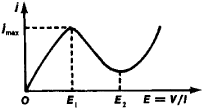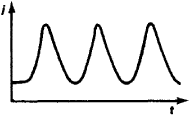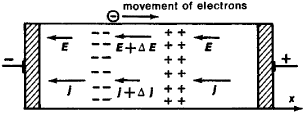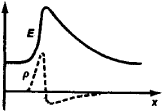# Gunn Effect

Also found in: Dictionary, Wikipedia.
Related to Gunn Effect: IMPATT diode, Gunn Oscillator

## Gunn effect

[′gən i‚fekt]
(electronics)
Development of a rapidly fluctuating current in a small block of a semiconductor (perhaps n-type gallium arsenide) when a constant voltage above a critical value is applied to contacts on opposite faces.
McGraw-Hill Dictionary of Scientific & Technical Terms, 6E, Copyright © 2003 by The McGraw-Hill Companies, Inc.
The following article is from The Great Soviet Encyclopedia (1979). It might be outdated or ideologically biased.

## Gunn Effect

a phenomenon in which high-frequency oscillations of electrical current j are generated in a semiconductor having an N-shaped spatial current-voltage characteristic (see Figure 1). The effect was first discovered by the American physicist J. Gunn in 1963 in two semiconductors having electron conductivity—gallium arsenide (GaAs) and indium phosphide (InP). Oscillations occur when a constant voltage V applied to a semiconductor specimen of length l is such that the electrical field E in the specimen, which is equal to E = V/l, is enclosed within certain limits E1EE2, where E1 and E2 are the boundaries of a falling section of the current-voltage characteristic j(E)—that is, where the differential resistance is negative. The current oscillations are in the form of pulses (see Figure 2). Their repetition frequency is inversely proportional to the length l of the specimen.Figure 1. N-shaped current-voltage characteristic: E is the electrical field created by the applied potential difference V; j is the current density

The Gunn effect is associated with the so-called electrical domain—a region of a strong electrical field—that periodically arises in the specimen, moves along it, and then disappears. The domain occurs because a uniform electric field is unstable where a negative differential resistance exists. In fact, let a nonhomogeneous distribution of the electron concentration in the form of a dipole layer arise at random in a semiconductor—in one region the electron concentration increases, and in the other it decreases (Figure 3). An auxiliary field ΔE appears between these charged regions (as it does between the plates of a charged capacitor). When this field is added to the external field E and the differential resistance of the specimen is positive—that is, when the current increases as the field E increases—then the current within the layer is larger than outside of it (Δj > 0). Therefore, electrons from the region of higher density flow out in greater amounts than they flow in, so that developing inhomogeneity is reabsorbed. However, if the differential resistance is negative (if the current decreases as the field increases), the current density is then smaller where the field is greater—that is, within the layer. The inhomogeneity that developed initially is not reabsorbed but, on the contrary, increases. The voltage drop increases across the dipole layer but decreases outside of it, because the total voltage on the specimen is fixed. Finally an electrical domain is created; its field and charge-density distributions are shown in Figure 4. The field outside of the established domain is smaller than the threshold E1; therefore, new domains do not develop.Figure 2. Shape of current oscillations in an instance of the Gunn effect

Since a domain is made up of current carriers—conduction electrons—it moves in the direction of their drift at a velocity v close to the drift velocity of the carriers outside the domain. Usually a domain develops at the cathode rather than within a specimen. Upon reaching the anode the domain disappears. As it disappears, the voltage drop diminishes across the domain and increases correspondingly in all other parts of the specimen. At the same time, the current in the specimen increases because the field outside of the domain is increasing; as this field approaches the threshold value El, the current density approaches a maximum jmax (see Figure 1). When the field outside of the domain exceeds E1, a new domain begins to form at the cathode, the current decreases, and the process is repeated. The frequency v of the current oscillations is equal to the reciprocal of the time required for a domain to pass through the specimen: v = v/l. In this respect the Gunn effect is essentially different from the generation of oscillations in other devices with an N-shaped current-voltage characteristic—for example, a tunnel diode, in which generation is not associated with the formation and movement of domains and the oscillation frequency is determined by the circuit capacitance and inductance.Figure 3. Development of an electrical domain. Electrons are moving from left to right against the field E.

For GaAs with an electron conductivity at room temperature E1 ~ 3 × 103 volts per cm, the domain velocity v ≈ 107 cm/sec. Specimens with length l = 50-300 µm are usually used; therefore, the oscillations generated have a frequency v = 0.3-2.0 gigahertz. The domain size is ~ 10-20 µm. The Gunn effect has been observed, in addition to GaAs and InP, in other electron semiconductors such as Ge, CdTe, ZnSe, and InSb, as well as in Ge with hole conductivity. It is utilized to make generators and amplifiers in the superhigh-frequency range.Figure 4. Distribution of the electrical field E (solid curve) and the space charge ρ (broken curve) in an electrical domain

### REFERENCES

Solid State Communications, 1963, vol. 1, no. 4, pp. 88-91.
Gunn, J. “Effekt Ganna.” Uspekhi fizicheskikh nauk, 1966, vol. 89, issue 1, p. 147.
Volkov, A. F., and Sh. M. Kogan. “Fizicheskie iavleniia v poluprovodnikakh s otritsatel’noi differentsial’noi provodimost’iu.” Uspekhi fizicheskikh nauk, 1968, vol. 96, issue 4, p. 633.
Levinshtein, M. E. “Effekt Ganna.” Zarubezhnaia radioelektronika, 1968, no. 10, p. 64.
Levinshtein, M. E., and M. S. Shur. “Pribory na osnove effekta Ganna.” Zarubezhnaia radioelektronika, 1970, no. 9, p. 58.

A. F. VOLKOV and SH. M. KOGAN